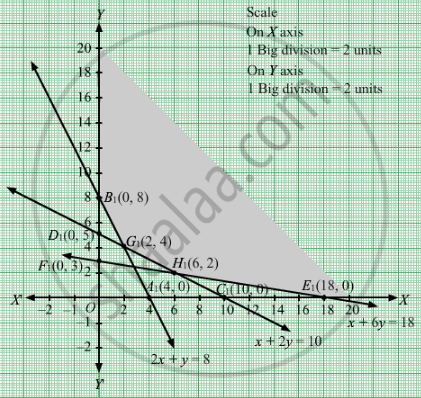# A Wholesale Dealer Deals in Two Kinds, a and B (Say) of Mixture of Nuts. Each Kg of Mixture a Contains 60 Grams of Almonds, 30 Grams of Cashew Nuts and 30 Grams of Hazel Nuts. - Mathematics

A wholesale dealer deals in two kinds, A and B (say) of mixture of nuts. Each kg of mixture A contains 60 grams of almonds, 30 grams of  cashew nuts and 30 grams of hazel nuts. Each kg of mixture B contains 30 grams of almonds, 60 grams of cashew nuts and 180 grams of hazel nuts. The remainder of both mixtures is per nuts. The dealer is contemplating to use mixtures A and B to make a bag which will contain at least 240 grams of almonds, 300 grams of cashew nuts and 540 grams of hazel nuts. Mixture A costs Rs 8 per kg. and mixture B costs Rs 12 per kg. Assuming that mixtures A and B are uniform, use graphical method to determine the number of kg. of each mixture which he should use to minimise the cost of the bag.

#### Solution

Let kg of kind A and y kg of kind B were used.
Quantity cannot be negative.
Therefore,

$x, y \geq 0$

The given information can be tabulated as follows:

 Nut Almonds(grams) Cashewnuts(grams) Hazel nuts(grams) A(x) 60 30 30 B(y) 30 60 180 Availability 240 300 540

Therefore, the constraints are

$60x + 30y \geq 240$
$30x + 60y \geq 300$
$30x + 180y \geq 540$

Mixture A costs Rs 8 per kg. and mixture B costs Rs 12 per kg.
Total cost  = Z = $8x + 12y$

which is to be minimised.
Thus, the mathematical formulat​ion of the given linear programmimg problem is
Min Z =  $8x + 12y$ subject to

$2x + y \geq 8$
$x + 2y \geq 10$
$x + 6y \geq 18$

$x, y \geq 0$

First, we will convert the given inequations into equations, we obtain the following equations:
2x y = 8, x +2y = 10, x +6y = 18, x = 0 and y = 0

Region represented by 2x y ≥ 8:
The line 2x y = 8 meets the coordinate axes at A1(4, 0) and B1(0, 8) respectively. By joining these points we obtain the line 2x y = 8.
Clearly (0,0) does not satisfies the inequation 2x y ≥ 8. So,the region in xy plane which does not contain the origin represents the solution set of the inequation 2x y ≥ 8.

Region represented by x +2y ≥ 10:
The line x +2y = 10 meets the coordinate axes at C1(10,0) and D1(0, 5) respectively. By joining these points we obtain the line
x +2y = 10. Clearly (0,0) does not satisfies the inequation x +2y ≥ 10. So,the region which does not contain the origin represents the solution set of the inequation x +2y ≥ 10.

Region represented by x +6y ≥ 18:
The line x +6y = 18 meets the coordinate axes at E1(18,0) and F1(0, 3) respectively. By joining these points we obtain the line x + 6y = 18.Clearly (0,0) does not satisfies the inequation x + 6y ≥ 18. So,the region which does not contain the origin represents the solution set of the inequation x +6y ≥ 18.

Region represented by x ≥ 0 and y ≥ 0:
Since, every point in the first quadrant satisfies these inequations. So, the first quadrant is the region represented by the inequations x ≥ 0, and ≥ 0.

The feasible region determined by the system of constraints 2x y ≥ 8, x + 2y ≥ 10,x + 6y ≥ 18, x ≥ 0, and y ≥ 0, are as follows.The corner points are B1(0, 8), G1(2, 4), H1(6, 2) and E1(18, 0).
The values of Z at these corner points are as follows

 Corner point Z= 8x + 12y B1 96 G1 64 H1 72 E1 144

The minimum value of Z is 64 which is attained at G1

$\left( 2, 4 \right)$ .

Thus, the minimum cost is Rs 64 obtained when 2 units of kind A and 4 units of kind B nuts were used.

Concept: Graphical Method of Solving Linear Programming Problems
Is there an error in this question or solution?

#### APPEARS IN

RD Sharma Class 12 Maths
Chapter 30 Linear programming
Exercise 30.3 | Q 8 | Page 39